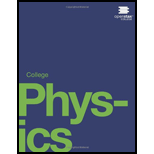# Professional Application (a) Calculate the maximum rate at which a rocket can expel gases if its acceleration cannot exceed seven times that of gravity. The mass of the rocket just as it runs out of fuel is 75,000-kg, and its exhaust velocity is 2.40 × 10 3 m/s. Assume that the acceleration of gravity is the same as on Earth's surface (9.80 m/s 2 ). (b) Why might it be necessary to limit the acceleration of a rocket?### College Physics

1st Edition
Paul Peter Urone + 1 other
Publisher: OpenStax College
ISBN: 9781938168000

#### Solutions

Chapter
Section### College Physics

1st Edition
Paul Peter Urone + 1 other
Publisher: OpenStax College
ISBN: 9781938168000
Chapter 8, Problem 58PE
Textbook Problem
1 views

## Professional Application(a) Calculate the maximum rate at which a rocket can expel gases if its acceleration cannot exceed seven times that of gravity. The mass of the rocket just as it runs out of fuel is 75,000-kg, and its exhaust velocity is 2.40 × 10 3 m/s. Assume that the acceleration of gravity is the same as on Earth's surface (9.80 m/s2). (b) Why might it be necessary to limit the acceleration of a rocket?

To determine

(a)

The maximum rate at which the rocket can expel gases

### Explanation of Solution

Given:

Mass of rocket m=75000kg

Exhaust velocity vg=2.40×103m/s

Maximum acceleration rate dvdt=8g

Let us consider the maximum expel rate be dmdt

Formula used:

We know the acceleration equation for rocket is given as

dvdt=dm

To determine

(b)

The reason why it is necessary to limit the acceleration of the rocket

### Still sussing out bartleby?

Check out a sample textbook solution.

See a sample solution

#### The Solution to Your Study Problems

Bartleby provides explanations to thousands of textbook problems written by our experts, many with advanced degrees!

Get Started

Find more solutions based on key concepts
What are the strengths and weaknesses of vegetarian diets?

Understanding Nutrition (MindTap Course List)

A slice of peach pie supplies 357 calories with 48 units of vitamin A; one large peach provides 42 calories and...

Nutrition: Concepts and Controversies - Standalone book (MindTap Course List)

Draw the structural formula for the carboxylate ion of propanoic acid.

Chemistry for Today: General, Organic, and Biochemistry

Are the two headlights of a car wired (a) in series with each other, (b) in parallel, or (c) neither in series ...

Physics for Scientists and Engineers, Technology Update (No access codes included)

Why do we see relatively few impact craters on Earth?

Oceanography: An Invitation To Marine Science, Loose-leaf Versin

Apart from food, list five things in your home that are homogeneous.

Introductory Chemistry: An Active Learning Approach